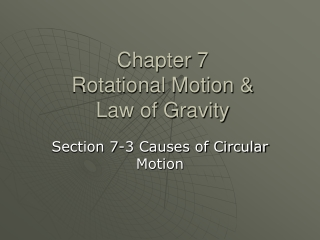DownloadDownload PresentationChapter 7 Rotational Motion & Law of Gravity

# Chapter 7 Rotational Motion & Law of Gravity

Download Presentation## Chapter 7 Rotational Motion & Law of Gravity

- - - - - - - - - - - - - - - - - - - - - - - - - - - E N D - - - - - - - - - - - - - - - - - - - - - - - - - - -
##### Presentation Transcript

1. Chapter 7Rotational Motion & Law of Gravity Section 7-3 Causes of Circular Motion

2. Force that Maintains Circular Motion Is: • The net force on an object directed toward the center of the object’s circular path. • Necessary for circular motion. • Measured in SI units of Newtons (N) • Fc

3. Figure 7-10 : When a ball is whirled in a circle, a force directed toward the center of the ball’s circular path acts on it.

4. Formulas for Fc • Fc = mvt2 / r • Force that maintains circular motion = mass x (tangential speed)2 / distance to axis • Fc = mrω2 • Force that maintains circular motion = mass x distance to axis x (angular speed)2

5. Practice Problem • Pg. 261 Sample 7H

6. Newton’s Universal Law of Gravitation • Gravitational force is a field force that always exists between two masses, regardless of the medium that separates them. • Gravitational force (Fg) depends on the distance between two masses. • The force between two masses decreases as the masses move farther apart.

7. Formula for Fg • Fg = G m1m2 / r2 • Gravitational Force = Constant x mass 1 x mass 2 / (distance between center of masses)2 • G is a universal constant = 6.673 x 10 -11 • Units = N∙m2 / kg2

8. Practice Problem • Pg. 264 Sample 7I

9. Assignment • Practice 7H pg. 261 #1-4 • Practice 7I pg. 265 # 1-3